Data analysts don’t count sheep at night. We look for a nice, bell-shaped curve in their arc as they leap over the fence. That’s normal distribution, and it’s a starting point to understanding one of the most important concepts in statistical analysis: the central limit theorem.

## Normal data? Nonnormal data? Look for a Pattern in the Distribution

Normal distribution of data follows a bell-shaped, symmetric pattern. Most observations are close to the average, and there are fewer and fewer observations going further from the average. It shows us that there is some method to the madness of the raw data.

If you liked thinking about those jumping sheep, artist Shuyi Chiou put together an even more imaginative example involving rabbits and the wingspan of dragons. It’s a great primer on several concepts that build off one another – sample size, distribution and the central limit theorem:

From rabbit size to rolling dice, the data from many scenarios follow a normal distribution. However, many things we would like to measure don’t follow this pattern. They are said to have a nonnormal distribution.

However for both normal AND nonnormal data, if we repeatedly take independent random samples of size n from a population, then when n is large, the distribution of the sample means will approach a normal distribution.

## How Large a Sample Size is Large Enough?

Well, it depends. The closer the population distribution already is to a normal distribution, the fewer samples you need to take to demonstrate the theorem. Generally speaking, a sample size of 30 or more is considered to be large enough for the central limit theorem to take effect. Populations that are heavily skewed or have several modes may require larger sample sizes, though.

### Related: How Much Data Do You Really Need?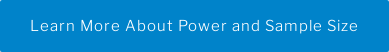## Example 1: Rolling a Die Shows Normal Distribution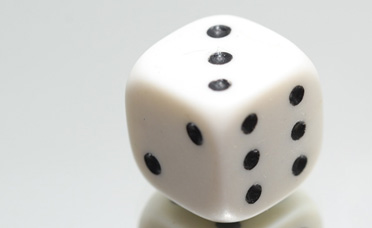Say you have a 6-sided die. The probability of rolling any of the numbers is 1/6. You have just as much probability of rolling any one number as you have of rolling the other five.

In Minitab Statistical Software you can take advantage of the random data generator to simulate 500 different outcomes for your first roll of the die. Click Calc > Random Data > Integer… and have it generate 500 rows where the minimum value is 1 and the maximum value is 6.

A histogram can be used to visualize those 500 “first rolls.” In this scenario, the sample size is 1. And because the odds of rolling each number are equal, the distribution is relatively flat. See how the blue bars in the graph below compare to the red curve representing normal distribution? It’s not normal.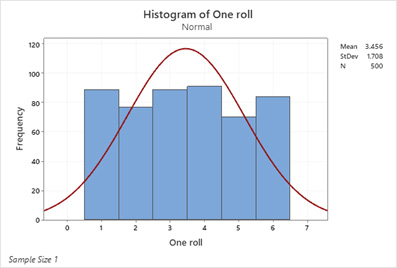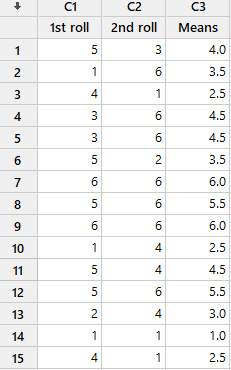Now, let’s take more samples and see what happens to the histogram of the averages of those samples. This time we will simulate rolling the die twice and repeating this process 500 times. Now the sample size is 2. We use Calc > Row Statistics… to compute the average of each pair. See below.

Here each row represents that sample of size 2 and its mean. When the sample size is large enough, this is going to follow a normal distribution. Let’s create a histogram of the means to get an idea.

It’s starting to look more normal.

Now, let’s roll the die 5, 10, 20 and 30 times.

The histograms for each set of means show that as the sample size increases, the distribution of sample means comes closer to normal.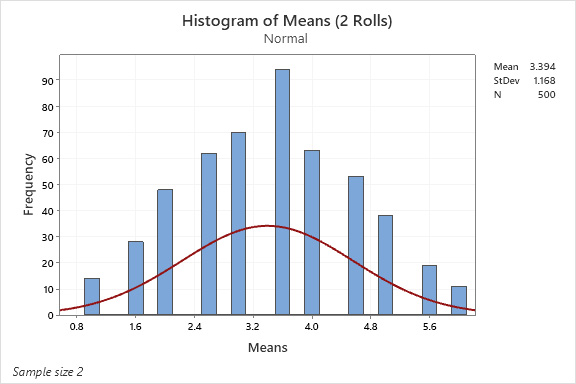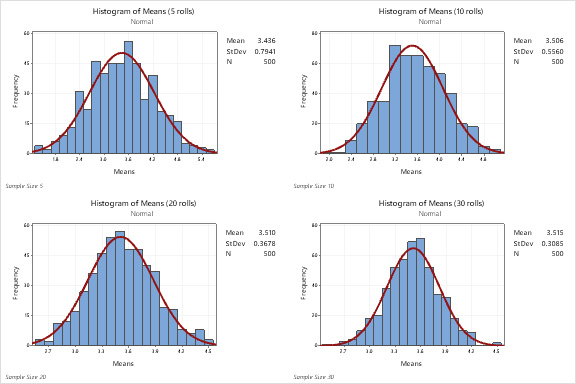### Related: Identifying the Distribution of Your Data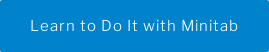## Example 2: Exponential Distribution

The exponential distribution models the time between events. It is a good model for the phase of a product or item's life when it is just as likely to fail at any time, regardless of whether it is brand new, a year old, or several years old (in other words, the phase before it begins to age and wear out during its expected application).

### Related: How a Poor Memory Helps to Model Failure Data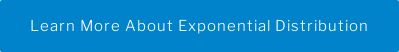Here’s an example of a probability density curve for the estimated time to failure of a transistor.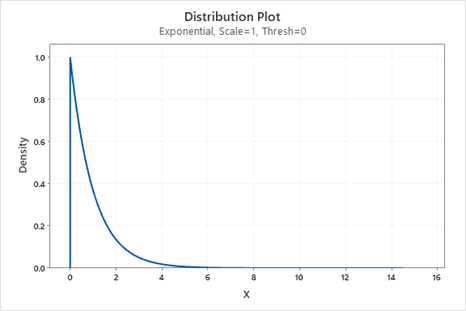Clearly, this is not a normal distribution. But what happens when you generate exponential data using a sample size of 5, calculate the means, and then create a histogram of the means? How about sample size 10, 20 and 30?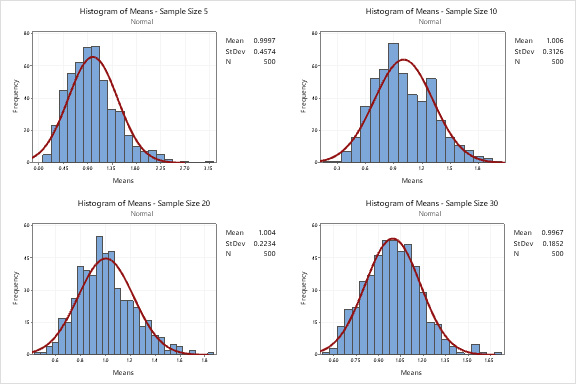Just like with rolling the die, the distribution of means more closely resembles the normal distribution as the sample size increases.

## Wrapping Up

Although it might not be frequently discussed by name outside of statistical circles, the Central Limit Theorem is an important concept. With demonstrations from dice to dragons to failure rates, you can see how as the sample size increases the distribution curve will get closer to normal.